# What is Atomic Mass?

Joseph Comunale, John Williams
• Author
Joseph Comunale

Joseph Comunale obtained a Bachelor's in Philosophy from UCF before becoming a high school science teacher for five years. He has taught Earth-Space Science and Integrated Science at a Title 1 School in Florida and has Professional Teacher's Certification for Earth-Space Science.

• Instructor
John Williams
What is Atomic Mass? See atomic mass examples, learn the atomic mass definition, how to find the atomic mass of an atom, and the importance of atomic mass. Updated: 08/15/2021

Show

## What is Atomic Mass?

Atoms are the smallest units of elements, or the simplest substances that cannot be broken down further chemically. Both atoms and elements are organized onto a display called the periodic table of elements.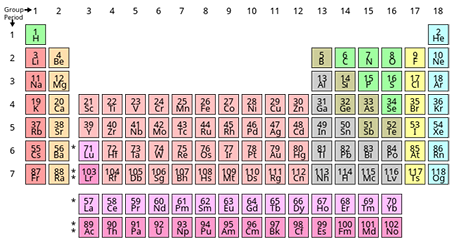Atoms are made of protons, neutrons, and electrons. The nucleus, or center of the atom that makes up most of the mass of the atom, is made up of protons and neutrons. Protons and neutrons are both hadron particles that have a positive charge or a neutral charge, respectively, and each are considered to have a mass of 1 atomic mass unit (amu). Amu's are units of measurement for atomic mass. It is not a coincidence that a proton and neutron are both equal to 1 amu. Atomic mass units were defined based on the masses of protons and neutrons by determining that one carbon atom has only six protons and usually six neutrons in its nucleus and therefore should be about 12 amu. This is much easier than saying one proton or neutron is equal to 1/167000000000000000000000th of a gram. Electrons are even smaller, and the mass of a proton is about 1,836 times greater than the mass of an electron.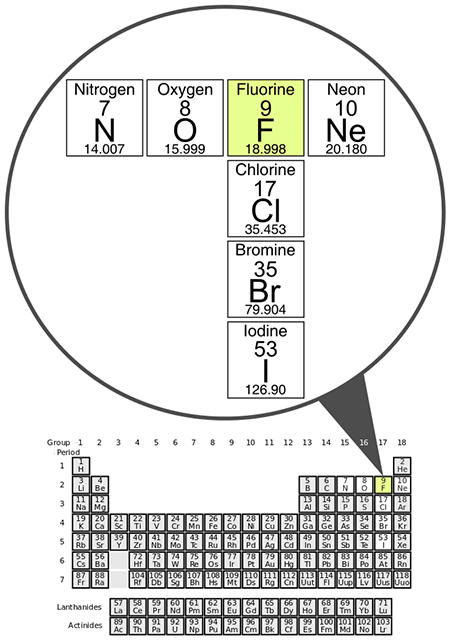Atomic mass is the total mass of particles of matter in an atom, i.e., the masses of protons, neutrons, and electrons in an atom added together. However, electrons are so small that they are negligible when finding the mass of an atom. Therefore, the atomic mass of an individual atom can be said to equal the total number of protons and neutrons. For example, fluorine atoms typically have 9 protons and 10 neutrons, and therefore have an atomic mass of 19 amu. When describing atoms and elements, there are three other numbers that may be present in a periodic table: atomic number, mass number, and average atomic mass.

### Atomic Number

The atomic number of an element is equal to the amount of protons that are contained in one atom of that element. Each element is made up of atoms that all have the same number of protons. For example, all carbon atoms have 6 protons, so carbon's atomic number is '6.'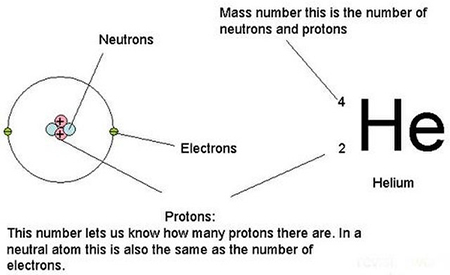### Mass Number

Mass number is the sum of the number of protons and neutrons in the nucleus of an atom. So, if an atom of carbon has 6 protons and 6 neutrons in its nucleus, its mass number will be exactly 12. When looking at the periodic table of elements, one can calculate the number of neutrons in at atom of a given element by using the following formula:

Number of neutrons = mass number - atomic number

So, carbon has an atomic number of 6 and a mass number of 12. Plugging those numbers into the formula allows one to determine that carbon atoms usually have 6 neutrons.

### Average Atomic Mass and Isotopes

When looking at a periodic table of elements, there is a number under each element that has decimal places. This is the average atomic mass of that element. Atoms of the same element always have the same number of protons but can vary in their number of neutrons. So, each element will have different isotopes, or atoms of the same element that have different numbers of neutrons. When looking at carbon on the periodic table, it says carbon has an average atomic mass of 12.011 amu. This number is determined by the fact that in any sample of carbon there are some atoms of carbon that have masses of 13 amu and 14 amu because carbon-13 atoms have 7 neutrons and carbon-14 atoms have 8 neutrons.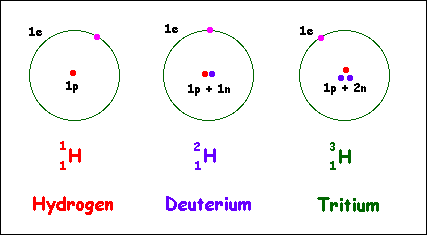### Atomic Mass Examples

Here are three examples of atomic mass and average atomic mass:

• The atomic mass of most boron atoms is about 11 amu, because of their 5 protons and 6 neutrons. The average atomic mass of boron is 10.81 amu because of the varying isotopes of boron.
• The atomic mass of most sodium atoms is about 23 amu, because of their 11 protons and 12 neutrons. The average atomic mass of oxygen is 22.99 amu because of the varying isotopes of sodium.
• The atomic mass of most copper atoms is about 63 amu, because of their 29 protons and 34 neutrons. The average atomic mass of copper is 63.55 amu because of the varying isotopes of copper.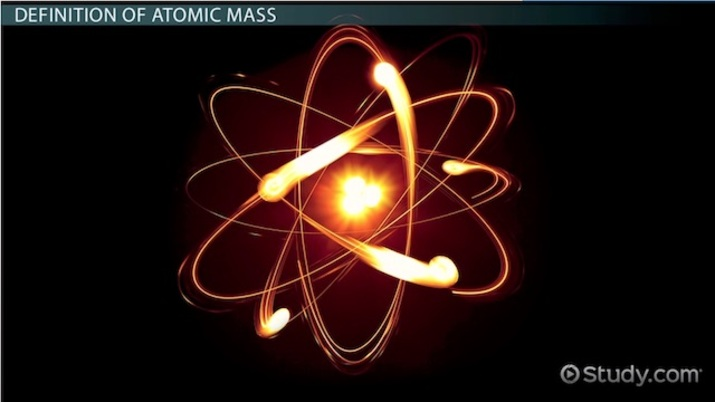An error occurred trying to load this video.

Try refreshing the page, or contact customer support.

Coming up next: What is Chromium? - Facts, Foods, Benefits & Side Effects

### You're on a roll. Keep up the good work!

Replay
Your next lesson will play in 10 seconds
• 0:01 Definition of Atomic Mass
• 1:15 Examples of Atomic Mass
• 2:02 Lesson Summar
Save Save

Want to watch this again later?

Timeline
Autoplay
Autoplay
Speed Speed

## How to Find the Atomic Mass of an Atom

One can find the atomic mass of an atom in a couple of ways. One way is to refer to the periodic table of elements. Usually, the atomic mass of an atom can be seen under an element's symbol and atomic number; it is commonly only found in the form of the average atomic mass or the mass number.

Another way to find the atomic mass of an atom is to add up the total mass of protons, neutrons, and electrons. But, because electrons are 1/1865th the mass of a proton or neutron, it is okay to simply add up the number of protons and the number of neutrons to get atomic mass. Adding up the masses of an atom's electrons when determining the mass is mostly only relevant when measuring the exact atomic mass differences between ions of the same element. Ions are atoms that are missing or have additional electrons when comparing them to the number of protons in the atom. To find the atomic mass of an element, one can look at the average atomic mass of an atom on the periodic table of elements.

## Importance of Atomic Mass

Atoms are too small to observe by shining light on them and seeing them under something like a microscope. Therefore, one of the only ways we can observe atoms and how they differ is through measuring their masses. Any sample or piece of something can be put into a mass spectrometer which will separate all the atoms by taking advantage of their different masses, and then provide the elemental composition of the sample.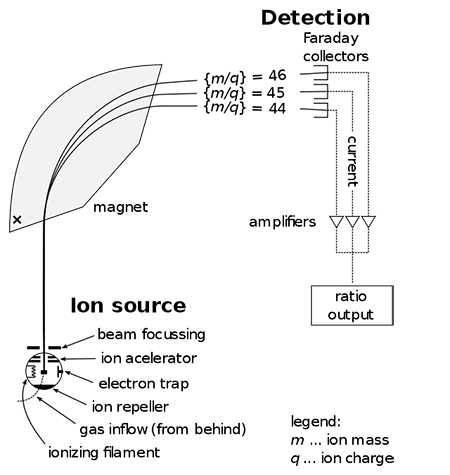To unlock this lesson you must be a Study.com Member.

#### How do we find atomic mass?

Atomic mass can be found by adding up the total masses of subatomic particles in an atom. Because both protons and neutrons have an atomic mass of 1 amu, and electrons have masses of such little consequence, atomic mass can be calculated by adding up the total number of protons and neutrons in an atom. Additionally, atomic mass can be found on the period table of elements.

#### What is the simple definition of atomic mass?

Atomic mass is the total mass of the subatomic particles in an atom and is measured in atomic mass units (amu).

### Register to view this lesson

Are you a student or a teacher?